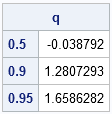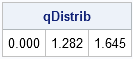The SAS/IML run-time library contains hundreds of functions and subroutines that you can call to perform statistical analysis. There are also many functions in Base SAS software that you can call from SAS/IML programs. However, one day you might need to compute some quantity for which there is no prewritten function.

Fortunately, the SAS/IML language enables you to define modules.

A module is a user-defined function or subroutine that you can call from an IML program. A module that returns a value is called a function module; a module that does not return a value is called a subroutine module. A subroutine module usually modifies one or more matrices that are passed in as arguments. You can call a subroutine module by using the CALL or RUN statement.

This post gives an example of defining a function module. A module enables you to encapsulate, reuse, and maintain related SAS/IML statements in a convenient way. You can pass matrices from your program into the module to provide input data and to control the way that the module behaves.

You define a module by using the START and FINISH statements. The START statement defines the name of the module and the arguments. You can use the RETURN statement to return a value from a function module.

For example, suppose you want to compute sample quantiles for a vector of values. You know that the UNIVARIATE procedure calculates sample quantiles, so you look up how to compute quantiles in the UNIVARIATE documentation. Several definitions are listed, but the default method for PROC UNIVARIATE is Definition #5. The following module definition is taken from the book Statistical Programming with SAS/IML Software:

```proc iml; /** Qntl: compute quantiles (Defn. 5 from the UNIVARIATE doc) **/ /** Arguments: q upon return, q contains the specified sample quantiles of the data. x is a matrix. The module computes quantiles for each column. p specifies the quantiles. For example, 0.5 specifies the median, whereas {0.25 0.75} specifies the first and third quartiles. This module does not handle missing values in the data. **/ start Qntl(q, x, p); /** definition 5 from UNIVARIATE doc **/ n = nrow(x); /** assume nonmissing data **/ q = j(ncol(p), ncol(x));/** allocate space for return values **/ do j = 1 to ncol(x); /** for each column of x... **/ y = x[,j]; call sort(y,1); /** sort the values **/ do i = 1 to ncol(p); /** for each quantile **/ k = n*p[i]; /** find position in ordered data **/ k1 = int(k); /** find indices into ordered data **/ k2 = k1 + 1; g = k - k1; if g>0 then q[i,j] = y[k2];/** return a data value **/ else /** average adjacent data **/ q[i,j] = (y[k1]+y[k2])/2; end; end; finish;```

After the module is defined, you can call it from your program. For example, the following statements compute the 50th, 90th, and 95th percentile of a vector of values sampled from the standard normal distribution:

```x = j(1000, 1); /** allocate 1000 rows and 1 column **/ call randseed(4321); /** set the random number seed **/ call randgen(x, "Normal");/** random sample from standard normal **/ p = {0.5 0.9 0.95}; /** specifies quantiles **/ call qntl(q, x, p); /** call module to compute sample quantiles **/ print q[rowname=(char(p))];```For comparison, you can use the QUANTILE function in Base SAS software to compute the quantiles of the standard normal distribution:

```/** quantiles of standard normal distribution **/ qDistrib = quantile("Normal", p); print qDistrib[format=6.3];```Because the data were sampled from a normal distribution, there is close agreement between the quantiles of the sample data (as computed by the QNTL module) and the quantiles of the standard normal distribution (as computed by the QUANTILE function).

Editor's Note: The QNTL function was included in SAS/IML 9.22 as built-in subroutine.

ShareDistinguished Researcher in Computational Statistics

Rick Wicklin, PhD, is a distinguished researcher in computational statistics at SAS and is a principal developer of SAS/IML software. His areas of expertise include computational statistics, simulation, statistical graphics, and modern methods in statistical data analysis. Rick is author of the books Statistical Programming with SAS/IML Software and Simulating Data with SAS.

1.Robert Pearson on

Will you give me some feedback on a related module I made? I needed to obtain percentiles of each column. The code below is what I came up with. Do you have any suggestions/improvements? Thanks,

start colPctls(k, x);
p = ncol(x);
n = nrow(x);
prob = k/100;
j = INT(n*prob);
g = n*prob - j;

out = j(p,1);
DO i=1 TO p;
CALL SORTNDX(ndx, x[,i], 1);
IF g=0 THEN
out[i] = (x[ndx[j], i] + x[ndx[j+1], i]) / 2;
ELSE
out[i] = x[ndx[j+1], i];
END;

RETURN(out);
finish;

2.Rick Wicklin on

Percentiles and quantiles are essentially the same thing, except that percentiles are measured on [0,100], and quantiles are measured on [0,1]. The 5th percentile is the 0.05 quantile, the 10th percentile is the 0.10 quantile, and so on. So just take your percentiles, divide then by 100, and then call the QNTL module like this:
pctl = {50 90 95};
call qntl(q, x, pctl/100);

3.Hi, I am having trouble with the sas/iml subroutine module. here is my code:

proc iml;
start corrp(a,b,c,d);
i= {1 2 3 4};
if p=i[1,a] & q=i[1,b] & s=i[1,c] & t=i[1,d] then

A=(y[p,q]-y[p,t]*y[q,t])/sqrt((1-y[p,t]*y[p,t])*(1-y[q,t]*y[q,t]));
B=(y[p,s]-y[p,t]*y[s,t])/sqrt((1-y[p,t]*y[p,t])*(1-y[s,t]*y[s,t]));
C=(y[q,s]-y[q,t]*y[s,t])/sqrt((1-y[q,t]*y[q,t])*(1-y[s,t]*y[s,t]));
rho=(A-B*C)/sqrt((1-B*B)*(1-C*C));

finish;

y={1 0.73456 0.71075 0.70398,0.73456 1.00000 0.69316 0.70855, 0.71075 0.69316 1.00000 0.83925, 0.70398 0.70855 0.83925 1.00000};
a=3;
b=4;
c=1;
d=2;
call corrp(3,4,1,2);
print rho;

my function is corrp(a,b,c,d), and I am trying to call the function with inputvalues a=3;b=4;c=1;d=2; however I keep getting the error message "Matrix has not been set to a value"

Any feedback would be highly appreciated. Thanks in advance!

•